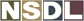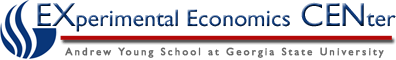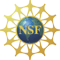Students / Subjects# The Quantity Theory of Money

The quantity equation of money relates the amount people hold to the transactions that take place.  This is expressed as:

M x V = P x T

M = the quantity of money

V = this is the rate that money will circulate in the economy

P = the price of a normal transaction

T = the number of times in a year that goods and services may be exchanged for money

This equation is important because it shows the relationship between transactions and money and what must change in order to keep the equation true if one of the variables change.  There are three assumptions that go along with this theory and they are that real output is fixed by the factors of production and therefore is independent of money supply, causation goes from money to prices, and that velocity is constant.